Jump to content

## Recommended Posts

Hi folks,

I am a beginner and interested in 3D environment.

I would like to create a scene that kind of shape that soft and marble geometry

But I don't know what kind of ways should I do for the scene.

Could you please recommend some way to get there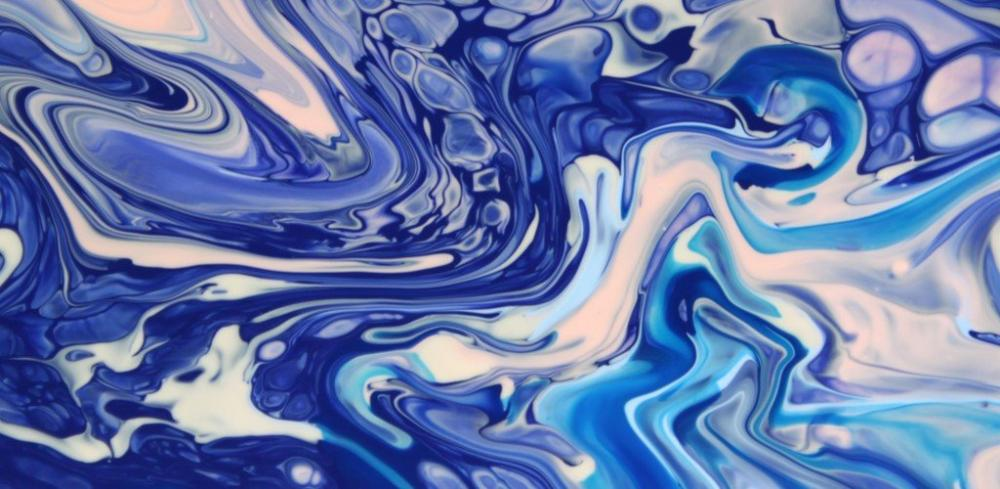Edited by wndyddl3
Edit title, added reference, grammar correct

#### Share this post

##### Share on other sites

@wndyddl3 Try This you have endless control. Than search how to make 2d fluid with Gas-solvers in Houdini and for the first you have Terrain Master Class in Houdini .And if you search on google you gonna find vex shaders /assets that you only need to copy paste ..plus you have that in ODForce forum already ,search Investigate share your Result .Have Fun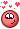```#define PI          3.1415926535897931

#pragma label       Theta       "Maximum Theta"
#pragma label       spread      "Overall Spread"
#pragma label       DATA        "Data Points"
#pragma label       kd          "Diffuse"
#pragma label       ks          "Specular"
#pragma label       bump        "Bump"

surface
qrotation_field
(   float   Theta   = 2.0,
spread  = 3,
kd      = 1,
ks      = 0.5,
bump    = 0.1;
string  DATA    = "\$HIP/../VEX/Reading-Data-Points/_datapoints1.bgeo.sc")
{
float d, Np, theta, pFratt, factor, pspread;
vector _P, pP, axis, pVratt;
vector4 Q;
string group;

_P = ptransform("space:camera", "space:world", P);

theta = snoise(_P + 0.9, 1, 0.75, 1)*4.5;
axis = snoise(_P + 0.05, 1, 0.05, 1);
Q = quaternion(theta, axis);
_P = qrotate(Q, _P);

for(int g = 0; g < 2; g++){
group = concat("group", itoa(g));
int p[] = expandpointgroup(DATA, group);
Np = len(p);
factor = g + 1;
for (int i = 0; i < Np; i++){
pP = point(DATA, "P", p[i]);
axis = point(DATA, "N", p[i]);
pFratt = point(DATA, "fratt", p[i]);
pVratt = point(DATA, "vratt", p[i]);
pspread = pFratt*spread/factor;
d = distance2(pP, _P);
d = exp(-d*d/pspread);
theta = 2*PI*(pFratt*2 - 1)*d*Theta/factor;
Q = quaternion(theta, axis);
_P = qrotate(Q, _P - pP) + pP;
}
}

vector outColor = snoise(_P*0.25, 1, 0.25, 1);

vector Nn = normalize(computenormal(P + min(outColor)*bump, N, Ng));

vector diff = diffuse(Nn)*outColor*dot(normalize(-I), N);

vector spec = specular(Nn, normalize(-I), fit01(min(outColor), 0.5, 0.1))*0.1;
spec += specular(N, normalize(-I), 0.05);

Cf = diff*kd + spec*ks;
}```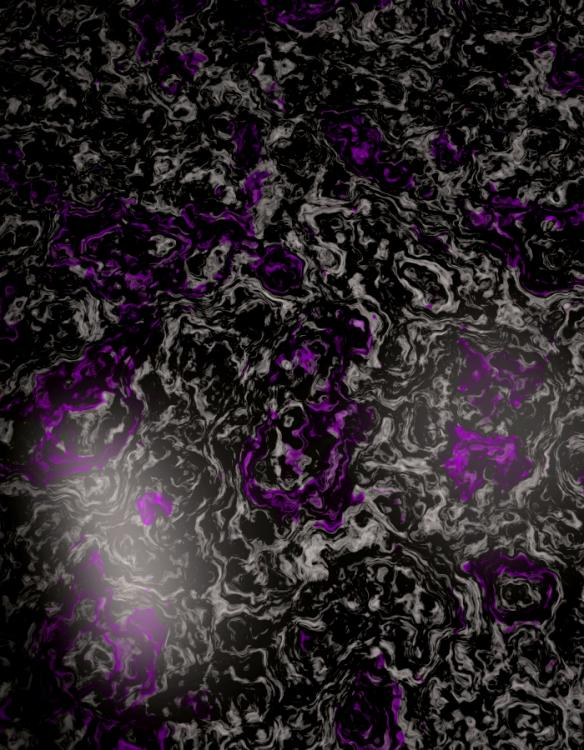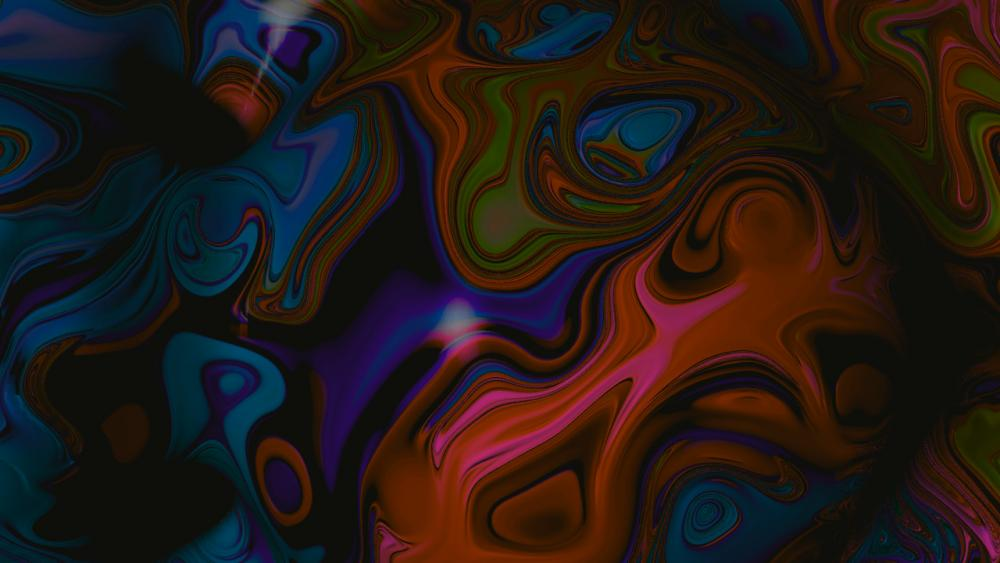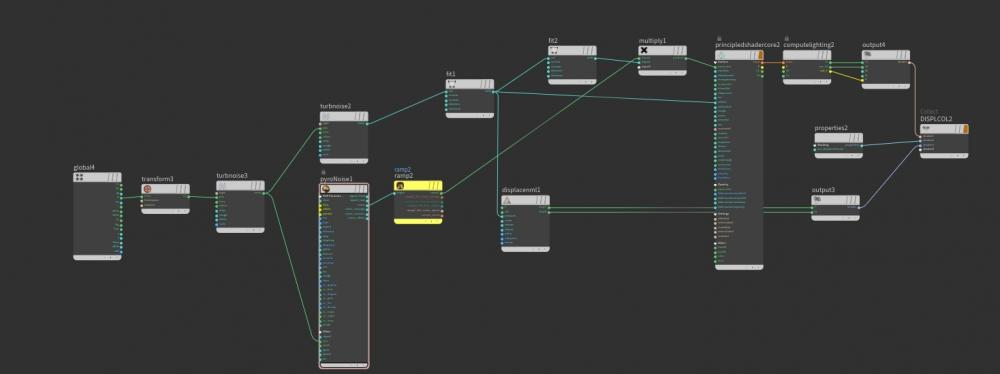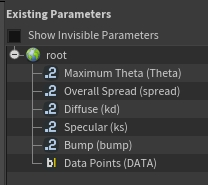•1

#### Share this post

##### Share on other sites

It's not exact what he posted, it's not blue and white.•1

#### Share this post

##### Share on other sites

Metal## Create an account or sign in to comment

You need to be a member in order to leave a comment

## Create an account

Sign up for a new account in our community. It's easy!

Register a new account

## Sign in

Already have an account? Sign in here.

Sign In Now

×
• Donations

• Leaderboard